# mW model of water

The mW model  of water is an atom with tetrahedrality intermediate between carbon and silicon. mW mimics the hydrogen-bonded structure of water through the introduction of a nonbond angular dependent term that encourages tetrahedral configurations. The model is given by: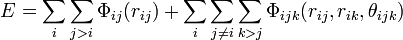$E = \sum_i \sum_{j>i} \Phi_{ij}(r_{ij}) + \sum_i \sum_{j\neq i} \sum_{k>j} \Phi_{ijk}(r_{ij}, r_{ik}, \theta_{ijk})$

where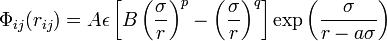$\Phi_{ij}(r_{ij}) = A \epsilon \left[ B \left(\frac{\sigma}{r} \right)^{p}- \left( \frac{\sigma}{r}\right)^q \right] \exp \left( \frac{\sigma}{r- a\sigma} \right)$

and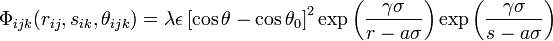$\Phi_{ijk}(r_{ij}, s_{ik}, \theta_{ijk}) = \lambda \epsilon \left[ \cos \theta - \cos \theta_0 \right]^2 \exp \left( \frac{\gamma \sigma}{r- a\sigma} \right) \exp \left( \frac{\gamma\sigma}{s- a\sigma} \right)$

where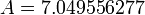$A = 7.049556277$,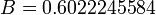$B = 0.6022245584$,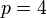$p = 4$,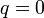$q = 0$, and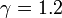$\gamma = 1.2$